## 基于高斯原理的非理想系统动力学建模$^{\bf 1)}$

*青岛理工大学理学院, 青岛市岩土力学与近海地下工程重点实验室, 青岛266520

## DYNAMIC MODELING OF NONIDEAL SYSTEM BASED ON GAUSS'S PRINCIPLE$^{\bf 1)}$

Yao Wenli,*,2), Liu Yanping*, Yang Liusong

*Qingdao Key Laboratory for Geomechanics and Offshore Underground Engineering, School of Science, Qingdao University of Technology, Qingdao 266520, China

College of Pipeline and Civil Engineering, China University of Petroleum, Qingdao 266580, China

 基金资助: 1)国家自然科学基金.  11272167

Received: 2019-03-5   Accepted: 2020-04-3   Online: 2020-07-18Abstract

The Gauss's principle gives the rules to identify the real motion from the possible motion by finding the extreme value of the function, which can make the dynamic problem of the multi-body system not need to solve the differential (algebra) equation, but adopt the optimization method of solving the minimum value, therefore, how to define the appropriate Gaussian constraint function is the prerequisite for the realization of dynamic optimization method. For the ideal system, the effect of constraints on the system can be reflected by the constraint equation and so the Gaussian constraint can be expressed as a function of the particle acceleration of the system, then the dynamic problem of the system can be described as the constrained optimization problem with the objective function as the Gaussian constraint function and the optimization variable as the particle acceleration. When the non-ideal factors such as dry friction need to be taken into account in the system, the partial interaction can not be covered by the defined constraint equation and needs to be described by additional physical laws. This sort of interaction destroys the extreme value characteristics of the original Gaussian restraint function of the system. Based on Gauss's principle of the variable classification, the extreme value principle of non-ideal system is derived and proved, whose objective function is expressed by ideal constraint forces. The Gauss's principle for non-ideal system in the existing literature is discussed and it is pointed out that it is an expression of the extreme value principle given when there is no obvious function correlation between the non-ideal constraint force and the ideal constraint force. But when they have obvious function relation (such as the linear relationship between the sliding friction force and the normal constraint force in the Coulomb friction law), this form will fail. And according to the extreme value principle given, the dynamic optimization model of contact problem for multi-body system considering friction is obtained. In the examples, the optimization model and the corresponding linear complementary model are analyzed, which is found that the two are necessary and sufficient conditions for each other under the condition of satisfying the uniqueness of rigid body sliding problem, thus, the reliability of the optimization model given is proved. And the dynamic simulation is carried out by the optimization calculation method. The simulation results show the feasibility and effectiveness of combining Gaussian principle with optimization algorithm.

Keywords： non-ideal ; multibody ; Gauss's principle ; optimization model

Yao Wenli, Liu Yanping, Yang Liusong. DYNAMIC MODELING OF NONIDEAL SYSTEM BASED ON GAUSS'S PRINCIPLE$^{\bf 1)}$. Chinese Journal of Theoretical and Applied Mechanics[J], 2020, 52(4): 945-953 DOI:10.6052/0459-1879-20-073

## 1 受约束系统高斯类动力学变分方程

### 1.1 笛卡尔坐标形式的高斯类变分方程

$\begin{eqnarray} \left.\begin{array}{l} {\varPhi }_1 ({u},t)={\bf0} \\ {\varPhi }_2 ({u},{\dot{{u}}},t)={\bf0} \\ {\varPsi }_1 ({u},t)\geqslant {\bf0} \\ {\varPsi }_2 ({u},{\dot{{u}}},t)\geqslant {\bf0} \\ \end{array}\right\} \end{eqnarray}$

$\begin{eqnarray}F^{Ci}=M'\ddot{u}-F-F^{Cn}\end{eqnarray}$

$\begin{eqnarray}(F^{Ci})^{\rm T}\delta \ddot{u}=(M'\ddot{u}-{F}-F^{Cn})^{\rm T}\delta \ddot{u}=0\end{eqnarray}$

### 1.2 广义坐标形式的高斯类变分方程

$\varPhi'_1 ({q},t)={\bf 0}$
$\varPhi'_2 ({q},\dot{{q}},t)={\bf 0}$
$\varPsi'_1 ({q},t)\geqslant{\bf 0}$
$\varPsi'_2 ({q},\dot{{q}},t)\geqslant{\bf 0}$

$A_1 ({q},{\dot{{q}}},t){\ddot{{q}}}={b}_1 ({q},{\dot{{q}}},t)$
$A_2 ({q},{\dot{{q}}},t)\ddot{{q}}\geqslant {b}_2 ({q},{\dot{{q}}},t)$

$\begin{eqnarray}{M\ddot{{q}}}={Q}+{Q}^{Ci}+{Q}^{Cn}\end{eqnarray}$

$\begin{eqnarray}{\ddot{{q}}}=M^{-1}{Q}+M^{-1}{GF}^{Ci}+M^{-1}{HF}^{Cn}\end{eqnarray}$

$\begin{eqnarray}{GF}^{Ci}={M\ddot{{q}}}-{Q}-{HF}^{Cn}\end{eqnarray}$

$\begin{eqnarray}(GF^{Ci})^{\rm T}\delta {\ddot{{q}}}=({M\ddot{{q}}}-{Q}-{HF}^{Cn})^{\rm T}\delta {\ddot{{q}}}=0\end{eqnarray}$

## 2 非理想系统极值形式的高斯原理的推导及证明

### 2.1 非理想系统高斯拘束及限制条件的推导

2.1.1 非理想约束力矢量与理想约束力无明显关联

$\begin{eqnarray}\delta {\ddot{{q}}}=M^{-1}G\delta F^{Ci}\end{eqnarray}$

$G_{ni} ({\ddot{{q}}})=\frac{1}{2}({M\ddot{{q}}}-{Q}-{Q}^{Cn})^{\rm T}M^{-1}({M\ddot{{q}}}-{Q}-{Q}^{Cn})\quad$
$G_{ni} (F^{Ci})=\frac{1}{2}(F^{Ci})^{\rm T}G^{\rm T}M^{-1}{GF}^{Ci}$

$\begin{eqnarray} \left.\begin{array}{l} {A}_1 M^{-1}{Q}+{A}_1 M^{-1}{FG}^{Ci}+{A}_1 M^{-1}{Q}^{Cn}={b}_1\\ {A}_2 M^{-1}{Q}+{A}_2 M^{-1}{GF}^{Ci}+{A}_2 M^{-1}{Q}^{Cn}\geqslant {b}_2 \\ \end{array}\right\} \end{eqnarray}$

2.1.2 非理想约束力矢量可以表达为理想约束力矢量的函数

$\begin{eqnarray} \left.\begin{array}{l} \delta {\ddot{{q}}}=M^{-1}(G+Hf_{Ci})\delta F^{Ci} \\ {f}_{Ci} =\dfrac{\partial {f}}{\partial F^{Ci}}\\ \end{array}\right\} \end{eqnarray}$

$\begin{eqnarray}(F^{Ci})^{\rm T}G^{\rm T}M^{-1}(G+Hf_{Ci})\delta F^{Ci}=0\end{eqnarray}$

$\begin{eqnarray}G_{ni} (F^{Ci})=\frac{1}{2}(F^{Ci})^{\rm T}G^{\rm T}M^{-1}(G+Hf_{Ci})F^{Ci}\end{eqnarray}$

$\begin{eqnarray} \left.\begin{array}{l} {A}_1 M^{-1}{Q}+{A}_1 M^{-1}{GF}^{Ci}+{A}_1 M^{-1}{f}(F^{Ci})={b}_1 \\ {A}_2 M^{-1}{Q}+{A}_2 M^{-1}{GF}^{Ci}+{A}_2 M^{-1}{f}(F^{Ci})\geqslant {b}_2 \\ \end{array}\right\} \end{eqnarray}$

$\begin{eqnarray}[{M\ddot{{q}}}-{Q}-{Y}({\ddot{{q}}})]^{\rm T}\delta {\ddot{{q}}}=0\end{eqnarray}$

### 2.2 非理想系统的高斯拘束取得最小值的条件及证明

$\Delta G_{ni} (F^{Ci})=\\ \qquad\frac{1}{2}(F^{Ci}+\Delta F^{Ci})^{\rm T}G^{\rm T}M^{-1}(G+Hf_{Ci})(F^{Ci}+\Delta F^{Ci}) -\\ \qquad\frac{1}{2}(F^{Ci})^{\rm T}G^{\rm T}M^{-1}(G+Hf_{Ci})(F^{Ci}) =\\ \qquad(\Delta F^{Ci})^{\rm T}G^{\rm T}M^{-1}(G+Hf_{Ci})(F^{Ci}) +\\ \qquad\frac{1}{2}(\Delta F^{Ci})^{\rm T}G^{\rm T}M^{-1}(G+Hf_{Ci})(\Delta F^{Ci}) =\\ \qquad\frac{1}{2}(\Delta F^{Ci})^{\rm T}G^{\rm T}M^{-1}(G+Hf_{Ci})(\Delta F^{Ci}) =\\ \qquad\frac{1}{2}(\Delta F^{Ci})^{\rm T}M^{-1}(G+Hf_{Ci})(\Delta F^{Ci}) =\\ \qquad\frac{1}{2}(\Delta F^{Ci})^{\rm T}G^{\rm T}M^{-1}(G+Hf_{Ci})(\Delta F^{Ci})$

$\begin{eqnarray} \left.\begin{array}{l} \min \left[\dfrac{1}{2}(F^{Ci})^{\rm T}G^{\rm T}M^{-1}(G+Hf_{Ci})F^{Ci}\right] \\\begin{array}{ll} {\rm s.t.}\ \ \ &{A}_1 M^{-1}{Q}+{A}_1 M^{-1}{GF}^{Ci}+{A}_1 M^{-1}{f}(F^{Ci})={b}_1 \\ &{A}_2 M^{-1}{Q}+{A}_2 M^{-1}{GF}^{Ci}+{A}_2 M^{-1}{f}(F^{Ci})\geqslant {b}_2 \\ \end{array} \end{array}\right\}\qquad \end{eqnarray}$

$\begin{eqnarray} \left.\begin{array}{l} \min \lt[\dfrac{1}{2}({M\ddot{{q}}}-{Q}-{Q}^{Cn})^{\rm T}M^{-1}({M\ddot{{q}}}-{Q}-{Q}^{Cn})] \\\begin{array}{ll} {\rm s.t.}\ \ & A_1 ({q},\dot{q},t)\ddot{{q}}={b}_1 ({q},{\dot{{q}}},t) \\ &A_2 ({q},\dot{q},t)\ddot{{q}}\geqslant {b}_2 ({q},{\dot{{q}}},t) \\ \end{array} \end{array}\right\}\quad \end{eqnarray}$

## 3 考虑库仑摩擦的单边约束的多体系统动力学的优化模型

$\begin{eqnarray}{g}_{\rm N} (q,t)\geqslant 0\end{eqnarray}$

${\dot{{g}}}_{\rm N} (q,t)={W}_{\rm N}{\dot{{q}}}+{\widetilde{{W}}}_{\rm N} \geqslant 0$
${\ddot{{g}}}_{\rm N} ({q},t)={W}_{\rm N}{\ddot{{q}}}+{\overline{{W}}}_{\rm N} \geqslant 0$

$\begin{eqnarray}{M\ddot{{q}}}={Q}+{W}_{\rm N}^{\rm T} {\lambda }+{W}_{\rm t}^{\rm T} F^{Cn}\end{eqnarray}$

$\begin{eqnarray}{\lambda}=\mbox{arg}\min \left[\frac{1}{2}(\lambda^*)^{\rm T}{A\lambda }^* \big| {A\lambda }^* +{B}\geqslant 0\right]\end{eqnarray}$

## 4 例子

### 图1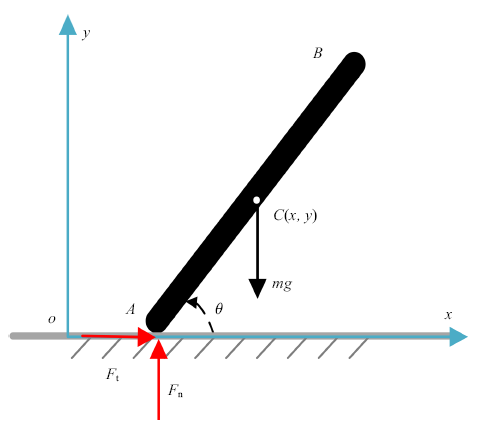Fig. 1   A rigid bar that slides along the ground

4.1.1 杆件运动学与动力学方程

$\begin{eqnarray} \left. \begin{array}{l} m\ddot{{x}}=F_{\rm t} \\ m\ddot{{y}}=F_{\rm n} -mg \\ \dfrac{1}{3}ml^2\ddot{{\theta }}=-F_{\rm n} l\cos \theta +F_{\rm t} l\sin \theta \\ \end{array} \right\} \end{eqnarray}$

$g_{\rm N} =y_A =y-l\sin \theta \geqslant 0$

$\ddot{{y}}=\ddot{{y}}_A +l\ddot{{\theta }}\cos \theta -l\dot{{\theta }}^2\sin \theta$

$\begin{eqnarray}\ddot{{y}}_A =A(\theta ,\mu )F_{\rm n} +B(\theta ,\dot{{\theta }})\end{eqnarray}$

$A=\frac{1}{m}[1+3\cos \theta (\cos \theta -\mu \sin \theta )]\\ B=l\dot{{\theta }}^2\sin \theta -g$

$M=\left[ {{\begin{array}{c} {m} & \\ & m & \\ && {\frac{1}{3}ml^2} \\ \end{array} }} \right], Q=\left[ {{\begin{array}{c} 0 \\ {-mg} \\ 0 \\ \end{array} }} \right]\\ {W}_{\rm N} =\left[ 0 \ 1\ {-l\cos\theta } \right] \ \ {W}_{\rm t} =\left[ 1 \ 0 \ {l\sin\theta } \right] \\ {s}=, \ \ {U}=\left[ \mu \right]$

${A}={W}_{\rm N} M^{-1}(W_{\rm N}^{\rm T} +{W}_{\rm t}^{\rm T} {sU})= [m^{-1}(1+3\cos^2\theta -3\mu\sin \theta \cos \theta )]=\left[A \right]\\ {B}={W}_{\rm N} M^{-1}{Q}+{\bar{{W}}}_{\rm N} =\left[ l\sin \theta \dot{\theta}^2-g\right]=\left[ B \right]$

4.1.2 接触模型及对比分析

(1)单边接触的线性互补模型 (linear complementary problem, LCP)

$\begin{eqnarray}F_{\rm n} \geqslant 0,\ \ \ddot{{y}}_A \geqslant 0,\ \ F_{\rm n} \cdot \ddot{{y}}_A =0\end{eqnarray}$

$\begin{eqnarray}F_{\rm n} \geqslant 0,\ \ AF_{\rm n} +B\geqslant 0,\ \ F_{\rm n}(AF_{\rm n} +B)=0\end{eqnarray}$

(2) 单边接触的高斯优化模型

$F_{\rm n} =\mbox{arg}\mathop {\min }\limits_{\lambda } \left( \frac{1}{2}(\lambda)^{\rm T}A{\lambda}|{A\lambda +B\geqslant 0}\right) = \mbox{arg}\mathop {\min }\limits_{\lambda } \left( \frac{1}{2}A{\lambda }^2| A\lambda +B\geqslant 0\right)$

(3) 两种模型之间的对比分析

① 由高斯优化模型可以推导得出LCP模型

$\begin{eqnarray} \lambda \geqslant 0,A\lambda +B\geqslant 0, \lambda (A\lambda +B)=0, \lambda(A\lambda +B)=0\ \ \ \end{eqnarray}$

② 由LCP模型可推出高斯优化模型

LCP模型与二次型优化问题紧密相连, 式(33)所代表的LCP模型可转化为二次型优化问题

$\begin{eqnarray}\mathop{\mbox{min}}\limits_{F_{\rm n} } \frac{1}{2}AF_{\rm n}^2 +BF_{\rm n},\ \ F_{\rm n} \geqslant 0\end{eqnarray}$

$\begin{eqnarray}\mathop {\mbox{min}}\limits_{F_{\rm n} } \frac{1}{2}AF_{\rm n}^2,\ \ AF_{\rm n} +B\geqslant 0\end{eqnarray}$

### 图2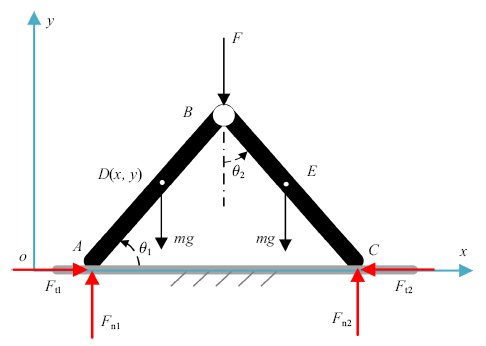Fig. 2   Two-bar system that slides along the ground

4.2.1 多点接触系统高斯优化模型

$\begin{eqnarray} \left.\begin{array}{l} g_{{\rm N}A} =y_A =y-\dfrac{l}{2}\sin \theta _1 \geqslant 0\\ g_{{\rm N}C} =y_C =y+\dfrac{l}{2}\sin \theta _1 -l\cos \theta _2 \geqslant 0 \\ \end{array}\right\} \end{eqnarray}$

$M= {{\begin{bmatrix} {2m} & 0 & {-\dfrac{1}{\mbox{2}}ml\sin \theta _1 } & {\dfrac{1}{\mbox{2}}ml\cos \theta _2 }\\ 0 & {2m} & {\dfrac{1}{\mbox{2}}ml\cos \theta _1 } & {\dfrac{1}{\mbox{2}}ml\sin \theta _2 }\\ {-\dfrac{1}{\mbox{2}}ml\sin \theta _1 } & {\dfrac{1}{\mbox{2}}ml\cos \theta _1 } & {\dfrac{1}{3}ml^2} & {-\dfrac{1}{\mbox{4}}ml^2\sin (\theta _1 -\theta _2 )}\\ {\dfrac{1}{\mbox{2}}ml\cos \theta _2 } & {\dfrac{1}{\mbox{2}}ml\sin \theta _2 } & {-\dfrac{1}{\mbox{4}}ml^2\sin (\theta _1 -\theta _2 )} & {\dfrac{1}{3}ml^2} \\ \end{bmatrix} }}$

$Q= {{\begin{bmatrix} 0 & F & {\dfrac{1}{2}Fl\cos \theta _1 } & 0 \\ \end{bmatrix} }} ^{\rm T} \\ {\bar{{W}}}_{\rm N} = {{\begin{bmatrix} {\dfrac{l}{2}\cos \theta _1 \dot{{\theta }}_1^2 +\dfrac{l}{2}\sin \theta _2 \dot{{\theta }}_2^2 } \\ {-\dfrac{l}{2}\sin \theta _1 \dot{{\theta }}_1^2 +\dfrac{l}{2}\cos \theta _2 \dot{{\theta }}_2^2 } \\ \end{bmatrix} }} \\ {W}_{\rm t} = {{\begin{bmatrix} 1 & 0 & {\dfrac{l}{2}\mbox{sin}\theta _1 } & 0 \\ 1 & 0 & {-\dfrac{l}{2}\mbox{sin}\theta _1 } & {l\mbox{cos}\theta _2 } \end{bmatrix} }} \\ {W}_{\rm N} = {{\begin{bmatrix} 0 & l & {-\dfrac{l}{2}\cos \theta _1 } & 0 \\ 1 & 0 & {\dfrac{l}{2}\mbox{sin}\theta _1 } & {l\sin \theta _2 } \\ \end{bmatrix} }} \\ {U}= {{\begin{bmatrix}{c} \mu & \\ & \mu \\ \end{bmatrix} }} ,\ \ \ {S}= {{\begin{bmatrix}{c} {sgn(\dot{{x}}_A )} & \\ & {sgn(\dot{{x}}_{\rm C} )} \\ \end{bmatrix} }}$

$\begin{eqnarray}{\lambda}=\mbox{arg}\min \left[ \frac{1}{2}(\lambda^*)^{\rm T}{A\lambda }^* | A\lambda ^* +B\geqslant 0 \right]\end{eqnarray}$

4.2.2 基于优化模型的动力学的计算过程及数值模拟

(1)输入初始条件${q}_0$, ${\dot{{q}}}_0$, 积分区间为$[0,T]$, 步长$\Delta t$以及系统的物理、几何参数;

(2)计算优化模型(39)中的各系数矩阵;

(3)计算法向约束力矢量${\lambda }$:

(4) 计算广义加速度矢量${\ddot{{q}}}$:

\begin{eqnarray}{\ddot{{q}}}=M^{-1}{Q}+M^{-1}{W}_{\rm N}^{\rm T} {\lambda }+M^{-1}{W}_{\rm t}^{\rm T} {SU\lambda }\end{eqnarray}

(5)根据步骤4所得到的广义加速度, 积分得到下一时刻广义坐标的速度和位移: $\dot{{q}}_{t+\Delta t}$, $q_{t+\Delta t}$;

(6)判断此时$t$是否满足终止条件$t\leqslant T$:

(a)如果满足, 程序终止并输出;

(b)如果不满足, 令$t=t+\Delta t$ 转到步骤2继续运行.

$m=2 \mbox{kg}, \ \ l=2 \mbox{m},\ \ \mu =0.2;\\ \theta _1 =\theta _2 =\frac{\pi}{4},\ \ x=y=l\sin \frac{\pi }{4} \mbox{m},\\ \dot{{x}}=-1 \mbox{m}/\mbox{s},\ \ \dot{{y}}=1 \mbox{m}/\mbox{s},\ \ \dot{{\theta }}_2 =0.1 {\mbox{rad}}/\mbox{s},\\ \dot{{\theta }}_1 =-0.1 {\mbox{rad}}/\mbox{s}.$

### 图3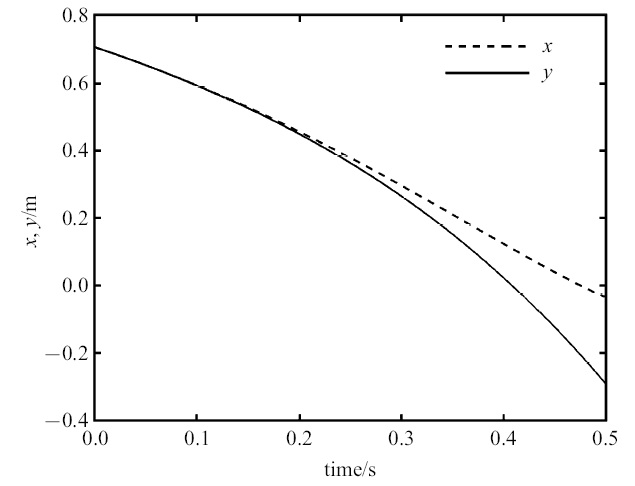Fig. 3   Time history of barycentric coordinates of bar $AB$

### 图4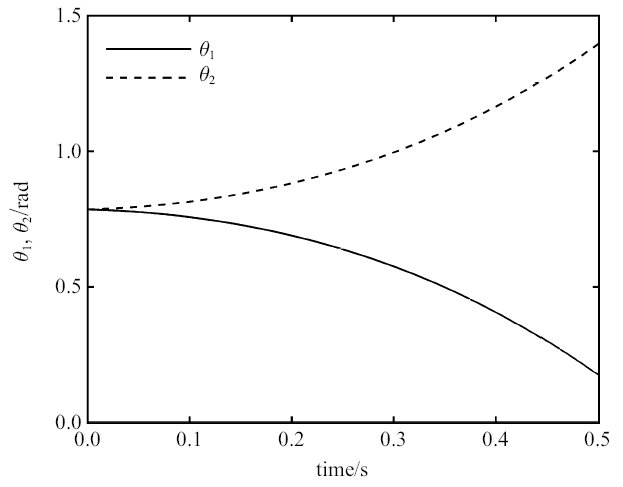Fig. 4   Time history of and rotation angles of bar $AB$

### 图5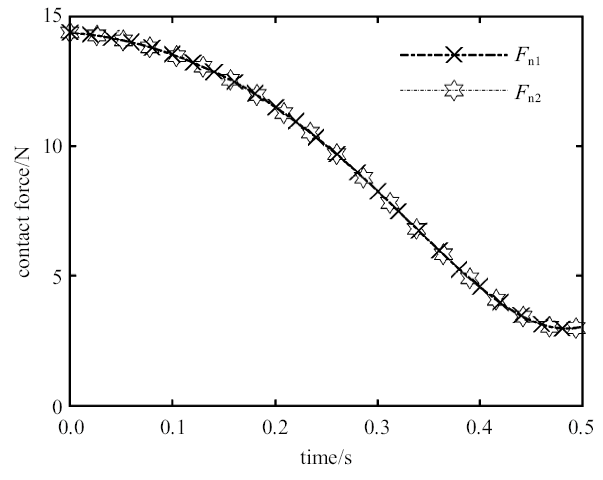Fig. 5   Time history diagram of normal binding force of contact point

## 5 结语

(1) 借助于高斯原理, 非光滑的多体系统动力学的求解过程可以从求解含互补条件的微分代数方程变为自然寻优的过程, 其寻优的过程非借助于互补性及最优化理论之间的关系, 而是使得目标函数的选择更加符合自然哲学的思想;

(2)因为原理本身所具有的极值形式可以将非理想系统的动力学问题引入求解优化问题的框架内, 从而可以尝试探索现代智能优化算法在非理想多体系统中的应用; 同时对于带控制的多体系统, 可以根据技术需要将动力学分析与系统的优化结合进行.

## 参考文献 原文顺序 文献年度倒序 文中引用次数倒序 被引期刊影响因子

// 第二十三届中国控制会议论文集, 2004: 356-358

( Ye Qingkai, Liu Caishan.

The discussion about using optimal control method solve mechanical problems

// Proceedings Of The 23rd China Control Conference, 2004: 356-358 (in Chinese))

( Liu Yanzhu, Pan Zhenkuan, Ge Xinsheng. Dynamics of Multibody Systems (2nd Edition). Beijing: Senior Education Press, 2014 (in Chinese))

Brogliato B.

Nonsmooth Mechanics: Models, Dynamics and Control (3rd edition)

London: Spinger-Verlag London Limited, 2016

Shabana AA.

An important chapter in the history of multibody system dynamics

Journal of Computational and Nonliear Dynamics, 2016,11(6):060303

( Hao Xiaoliang, Ge Zhaoqiang. Optimization and Optimal Control. Xi'an: Xi'an Jiaotong University Press, 2009 (in Chinese))

( Chen Bin. Analytical Dynamics. Beijing: Peking University Press, 2012 (in Chinese))

( Bobofu E $\Pi$. Dynamics And Algorithm of Operating Robot. Yu Liji, Chen Dunjie, transl. Beijing: Mechanical Industry Press, 1984 (in Chinese))

Lilov L, Lorer M.

Dynamic analysis of multirigid-body system based on the Gauss principle

ZAMM, 1982,62:539-545

( Liu Yanzhu.

Dynamic modeling of pole net system based on gauss principle

Journal of Dynamics and Control, 2018,16(4):289-294 (in Chinese))

URL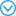Based on the generalized principles of dynamics, the feature of Gauss principle of least constraint is that the motion law can be directly obtained by using the variation method of seeking the minimal value of the constraint function without establishing any dynamic differential equations. According to the Kirchhoff's dynamic analogy, the configuration of an elastic rod can be described by the rotation of rigid cross section of the rod along the centerline. Since the local small change of the attitude of cross section can be accumulated infinitely along the arc-coordinate, the Kirchhoff's model is suited to describe the super-large deformation of elastic rod. Therefore the analytical mechanics of elastic rod with arc-coordinate s and time t as double arguments has been developed. The Cosserat model of elastic rod takes into consideration the factors neglected by the Kirchhoff model, such as the shear deformation of cross section, the tensile deformation of centerline, and distributed load, so it is more suitable to modeling a real elastic rod. In this paper, the model of the Cosserat rod is established based on the Gauss principle, and the constraint function of the rod is derived in the general form. The plane motion of the rod is discussed as a special case. As regards the special problem that different parts of the rod in space are unable to self-invade each other, a constraint condition is derived to restrict the possible configurations in variation calculation so as to avoid the invading possibility.

( Liu Yanzhu, Xue Yun.

Dynamic model of Coserat elastic rod based on Gauss principle

Acta Physica Sinica, 2015,64(4):44601 (in Chinese))

URLBased on the generalized principles of dynamics, the feature of Gauss principle of least constraint is that the motion law can be directly obtained by using the variation method of seeking the minimal value of the constraint function without establishing any dynamic differential equations. According to the Kirchhoff's dynamic analogy, the configuration of an elastic rod can be described by the rotation of rigid cross section of the rod along the centerline. Since the local small change of the attitude of cross section can be accumulated infinitely along the arc-coordinate, the Kirchhoff's model is suited to describe the super-large deformation of elastic rod. Therefore the analytical mechanics of elastic rod with arc-coordinate s and time t as double arguments has been developed. The Cosserat model of elastic rod takes into consideration the factors neglected by the Kirchhoff model, such as the shear deformation of cross section, the tensile deformation of centerline, and distributed load, so it is more suitable to modeling a real elastic rod. In this paper, the model of the Cosserat rod is established based on the Gauss principle, and the constraint function of the rod is derived in the general form. The plane motion of the rod is discussed as a special case. As regards the special problem that different parts of the rod in space are unable to self-invade each other, a constraint condition is derived to restrict the possible configurations in variation calculation so as to avoid the invading possibility.

URLBased on the Gauss's principle of least constraint, the dynamic modeling of a multi-body system connected by an elastic cable with varied lengths and large deformation in gravitational field of the earth was proposed in this paper. The practical background of the topic is the release process of a tethered satellite. The Kirchhoff's method was applied to transform the deformation of the elastic cable to rotation of rigid cross section along the centerline of the cable. Since the local small deformation of the cable can be accumulated limitlessly along the arc-coordinate, the Kirchhoff's model is suitable to describe the super-large deformation of elastic rod. In present paper the Gauss's constraint function of the system of rigid-flexible bodies in gravitational field of the earth was derived, and the geometric constraint conditions concerning relative position of bodies in space were considered using the Lagrange's multipliers. Therefore the dynamical model of the system was established in the form of conditional extremum problem. Applying the approach of Gauss's principle the real motion of the system can be obtained by the variation method directly through seeking the minimal value of constraint function without differential equations. The unified form of the model does not changed for different topologic constructions of the system, and it is unnecessary to distinct the tree system or system with loops. In the case of multi-body system with automatic control, the dynamic analysis can be combined with the optimization for different technique objectives.

( Liu Yanzhu.

Dynamic modeling of multibody system based on Gauss principle

Chinese Journal of Theoretical and Applied Mechanics, 2014,46(6):940-945 (in Chinese))

URLBased on the Gauss's principle of least constraint, the dynamic modeling of a multi-body system connected by an elastic cable with varied lengths and large deformation in gravitational field of the earth was proposed in this paper. The practical background of the topic is the release process of a tethered satellite. The Kirchhoff's method was applied to transform the deformation of the elastic cable to rotation of rigid cross section along the centerline of the cable. Since the local small deformation of the cable can be accumulated limitlessly along the arc-coordinate, the Kirchhoff's model is suitable to describe the super-large deformation of elastic rod. In present paper the Gauss's constraint function of the system of rigid-flexible bodies in gravitational field of the earth was derived, and the geometric constraint conditions concerning relative position of bodies in space were considered using the Lagrange's multipliers. Therefore the dynamical model of the system was established in the form of conditional extremum problem. Applying the approach of Gauss's principle the real motion of the system can be obtained by the variation method directly through seeking the minimal value of constraint function without differential equations. The unified form of the model does not changed for different topologic constructions of the system, and it is unnecessary to distinct the tree system or system with loops. In the case of multi-body system with automatic control, the dynamic analysis can be combined with the optimization for different technique objectives.

Moreau JJ.

Quadratic programming in mechanics: Dynamics of one-sided constraints

J. SIAM Control., 1966,4(1):153-158

URLApplying the Gauss minimum constraint theory，a finite segment modeling method is presented for a rigid-flexible coupled system. The cantilever beam’s movement with considerable range is synthesized in the model，and the system’s dynamic characteristic is expressed integrally. The simulation results and error analysis are given，which shows that the method has considerable preci?sion. The Gauss minimum constraint theory has its uniform expression，and it can reflect the system’s inertia effect. It is suitable for use in the modeling and analysis of a rigid- flexible coupled system.

( Dong Longlei, Yan Guirong, Du Yanting, et al.

The application of Gauss minimum restraint principle in the analysis of a kind of rigid flexible coupling system

Acta Armamentarii, 2001,22(3):347-351 (in Chinese))

URLApplying the Gauss minimum constraint theory，a finite segment modeling method is presented for a rigid-flexible coupled system. The cantilever beam’s movement with considerable range is synthesized in the model，and the system’s dynamic characteristic is expressed integrally. The simulation results and error analysis are given，which shows that the method has considerable preci?sion. The Gauss minimum constraint theory has its uniform expression，and it can reflect the system’s inertia effect. It is suitable for use in the modeling and analysis of a rigid- flexible coupled system.

( Hao Mingwang, Ye Zhengyin.

Gauss' principle of least constraint of single flexible body and multi-flexible body dynamics

Journal of Guangxi University $($Natural Science Edition$)$, 2011,36(2):195-204 (in Chinese))

Cosserat生长弹性杆动力学的 Gauss 最小拘束原理

( Xue Yun, Qu Jiale, Chen Liqun.

Gauss minimum restraint principle for the dynamics of Coserat growing elastic rod

Applied Mathematics and Mechanics, 2015,36(7):700-709 (in Chinese))

( Shi Yuedong, Wang Deshi.

Application of Gauss minimum constraint principle in gun vibration analysis

Journal of Gun Launch & Control, 2009,30(4):26-30 (in Chinese))

( Sun Jialiang, Tian Qiang, Hu Haiyan.

Advances in dynamic modeling and optimization of flexible multibody systems

Chinese Journal of Theoretical and Applied Mechanics, 2019,51(6):1565-1586 (in Chinese))

( Zhu An, Chen Li.

Mechanical simulation and full order sliding mode collision avoidance compliant control based on neural network of dual-arm space robot with compliant mechanism capturing satellite

Chinese Journal of Theoretical and Applied Mechanics, 2019,51(4):1156-1169 (in Chinese))

Feng Q, Tu J.

Modeling and algorithm on a class of mechanical systems with unilateral constraints

Arch. Appl. Mech., 2006,76:103-116

URLIn the present paper, the cable-structures are considered as a class of mechanical complementary-slackness systems. Based on the optimization algorithms used for multi-body dynamics with unilateral contacts, an algorithm by means of artificial neural network (NNW) is developed. The following two classes of cable-structures have been considered force-elongation of cable member follows elastic behavior and work-hardening assumption. Due to simplicity the former is used to prove the method reliability, and the latter, as general cable-structure problem is handled. First, the complementarity problems for those structures have been formulated; then using generalized Gaussian‘ least action principle they are summarized as an optimization problem. Based on Hopfield’s work, an artificial NNW has been designed and used to decide combination of possible constraints at each step in simulation. As examples, two cable-structures have been investigated. The calculated results for a simple suspension structure evidence the reliability and time-economization of the proposed method. An example of guyed mast shows the suitability of the proposed method for practical cable-structures.

Bruyninckx H, Khatib O.

Gauss principle and the dynamics of redundant and constrained manipulators

// Proceedings of the 2000 IEEE International Conference on Robotics & Automation, 2000: 2563-2569

A new perspective on constrained motion

Proc. R. Soc. Lond. A Math. Phys. Sci., 1992,439:407-410

Equations of motion for nonholonomic, constrained dynamical systems via Gauss's principle

Transactions of the ASME, 1993,60:662-668

Kalaba R, Natsuyama H, Udwadia F.

An extension of Gauss's principle of least constraint

Int J General Systems, 2004,33(1):63-69

Fan YY, Kalaba RE, Natsuyama HH, et al.

Reflections on the Gauss principle of least constraint

Journal of Optimization Theory and Applications, 2005,127(3):475-484

URLThe Gauss principle of least constraint is derived from a new point of view. Then, an extended principle of least constraint is derived to cover the case of nonideal constraints. Finally, a version of the principle for general underdetermined systems is adumbrated. Throughout, the notion of generalized inverses of matices plays a prominent role.

URLAnalytical mechanics is established based on d'Almbert-Lagrange Principle, Gauss principle, Jourdian principle and Hamilton principle, to deal with the dynamics of mechanical systems subject to holonomic or nonholonomic constraints. The governing equation of the systems are derived either by introducing Lagrange's multipliers to adjoin with the limitation equations for the virtual displacements, or by directly eliminating the constraint equations to achieve minimal formulations. The author presents a survey for the history of analytical mechanics, and explains some basic concepts, such as virtual displacement, ideal constraint, and the correlations between the Lagrange multipliers and the real constraint forces.

( Liu Caishan.

The fundamental equations in analytical mechanics for nonholonomic systems

Acta Scientiarum Naturalium Universitatis Pekinensis, 2016,52(4):756-766 (in Chinese))

URLAnalytical mechanics is established based on d'Almbert-Lagrange Principle, Gauss principle, Jourdian principle and Hamilton principle, to deal with the dynamics of mechanical systems subject to holonomic or nonholonomic constraints. The governing equation of the systems are derived either by introducing Lagrange's multipliers to adjoin with the limitation equations for the virtual displacements, or by directly eliminating the constraint equations to achieve minimal formulations. The author presents a survey for the history of analytical mechanics, and explains some basic concepts, such as virtual displacement, ideal constraint, and the correlations between the Lagrange multipliers and the real constraint forces.

Pfeiffer F.

Multibody Dynamics with Unilateral Contacts

United States of America: John Wiley and Sons. Inc, 1995

New general principle of mechanics and its application to general nonideal nonholonomic systems

Journal of Engineering Mechanics, 2005. 131(4):444-450

URL( Yao Wenli, Dai Baoqing.

Gauss principle of least constraint in generalized coordinates and its generalization

Mechanics in Engineering, 2014,36(6):779-785 (in Chinese))

URLURL&nbsp;By variational Gauss principle in the form of kinetic energy and explicit express, the meaning of items in Gauss constraints is confirmed. The optimization model of dynamics of multi-rigid-body system in the form of Descartes generalized coordinates is established based on Gauss principle of least constraint in generalized coordinates. The method to write the Gauss constraints in other coordinate system according to the above model is studied. The dynamical problem of multi-rigid-body system can be turned into a problem for a minimum, and provided by the relation between the Descartes generalized coordinates and other coordinates system, the Gauss constraint in this kind of coordinates system is easy to be obtained. The modeling method is more convenient and is of universality. The dynamical models are established for planar motion and fixed axis rotation by Descartes generalized coordinate and Lagrange coordinate, and the validity of this method is proved.

( Yao Wenli.

Dynamical modeling of multi-rigid-body system based on Gauss principle of least constraint in generalized coordinates

Acta Scientiarum Naturalium Universitatis Pekinensis, 2016,52(4):708-712 (in Chinese))

URL&nbsp;By variational Gauss principle in the form of kinetic energy and explicit express, the meaning of items in Gauss constraints is confirmed. The optimization model of dynamics of multi-rigid-body system in the form of Descartes generalized coordinates is established based on Gauss principle of least constraint in generalized coordinates. The method to write the Gauss constraints in other coordinate system according to the above model is studied. The dynamical problem of multi-rigid-body system can be turned into a problem for a minimum, and provided by the relation between the Descartes generalized coordinates and other coordinates system, the Gauss constraint in this kind of coordinates system is easy to be obtained. The modeling method is more convenient and is of universality. The dynamical models are established for planar motion and fixed axis rotation by Descartes generalized coordinate and Lagrange coordinate, and the validity of this method is proved.

URL( Yang Liusong, Yao Wenli, Yue Rong.

Simulation of body dynamics with optimization

Mechanics in Engineering, 2015,37(4):488-491 (in Chinese))

URL/

 〈〉